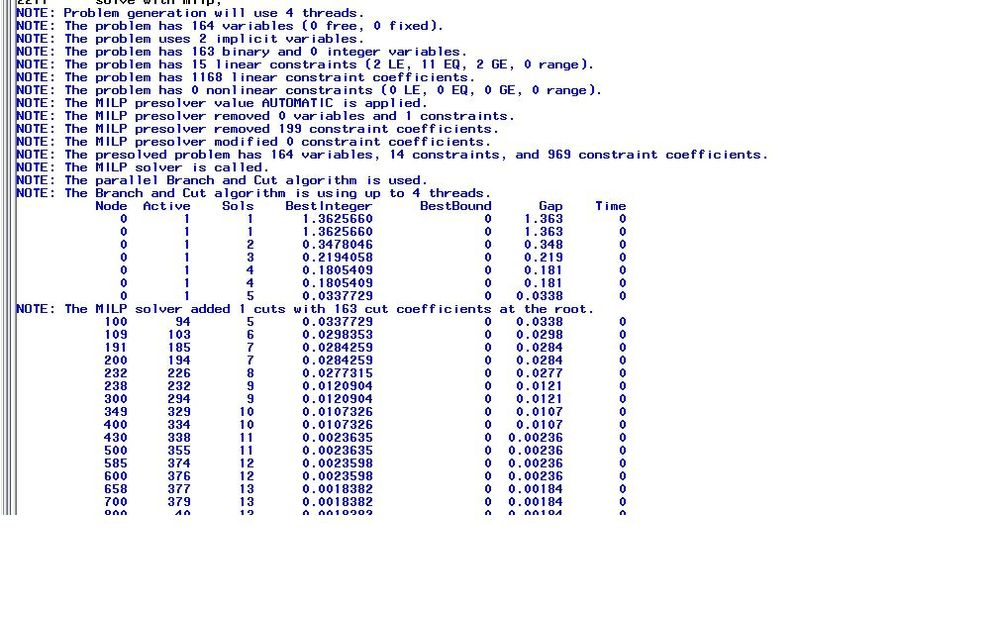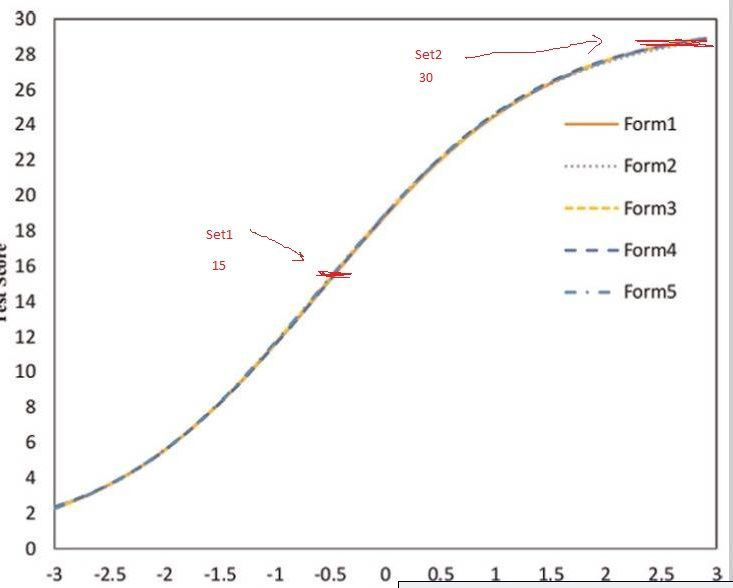## Proc OPTMODEL - using objective in 4 constraints causes 'out of memory'

Hello,

I'm using version 9.4 with about 8gb of free memory for SAS to use on this optimization problem - not sure if that's too small for my problem. This is my first time trying to use Proc OPTMODEL. I'm pretty familiar with base sas, but this method of thinking/coding is very new to me. I'm trying to use an automated test assembly method as detailed in van der linden "linear models for optimal test assembly" (image attached for section describing 5.18-5.21).BACKGROUND:

Essentially I have a pool of test items. Each item has categories it can belong to that I've marked in the "binary" dataset.

Content: addition, subtraction, fraction, multiplication, etc

Depth of knowledge: DOK1, DOK2, DOK3

Item type: multiple choice, constructed response, etc

Each item also has parameters that are associated with an item response theory model. When plugging these parameters into a function and adding all the item functions together you get a test function value. The goal of the automated test assembly is to match a target test function with the sum of these optimally selected item function values, while also hitting specific content , depth of knowledge, and item type constraints.

In my data I have the target values as 'set1' and 'set2', and I have the item function values that I've already calculated at specific x axis points as r1 and r2. The r1s for selected items should sum as close as possible to the value of 'set1'. The main constraints that are causing problems are below. The categorical constrains on content and such don't seem to be causing problems. When SAS runs out of memory, it still produces results that are basically what I want, which is what makes me believe that perhaps my coding method is just really inefficient:

con TCC1: total_r1 <= set1+y; /*minimizes positive deviations*/
con TCC2: total_r1 >= set1-y; /*minimizes negative deviations*/
con TCC3: total_r2 <= set2+y; /*minimizes positive deviations*/
con TCC4: total_r2 >= set2-y; /*minimizes negative deviations*/

This is basically saying I want to minimize the difference between target function and sum of the optmodel selected item functions at 2 points on the x axis (set1 and set2 are the target values of the y axis at 2 points on the x axis, r1 and r2 are sums of sas selected items for the 2 points on the x axis). I've attached a portion of the log. One thing I'm possibly concerned about is that it says, "The problem has 164 variables (0 free, 0 fixed)" and I'm guessing I'd want some that are free (but not sure). I've attached a really badly edited image of what the target function looks like, and what set1 and set2 might represent to maybe give a better visualization of what I'm trying to do, but it's a test of 30 items while the one I'm working with will have 40, but the idea is the same.

Any help or advice would be greatly appreciated. Thank you so much. PS: I can possibly post the data, but I'd have to get that approved so I'm trying without for now.Full OPTMODEL code is below:

```proc optmodel;
*begin the setup for the model - input all the data;
set theta_pts=1..2; *have 2 locations need to match;

set <str> BINARIES;

set <str> NUMERICS;

set <num> ITEMS;
str uin {ITEMS};
num binary {BINARIES, ITEMS} ; *tried labeling as var and binary, but when printing at end, does not populate with 1&0 only 0's;
num numeric {NUMERICS, ITEMS};
*assign var to make sure item is only assigned once per test;
var Assign {ITEMS,ITEMS} >=0;

*probably a better way to do this, but this seems to work;
{i in BINARIES} <binary[i,_N_]=col(i)>
{i in NUMERICS} <numeric[i,_N_]=col(i)>;

*model specification;
var x{ITEMS} BINARY, y >=0; *x is selection 1/0,  y is deviation;
min obj=y; *minimize deviation from target;

*target r1 r2 totals;
num set1=15;
num set2=30;

impvar total_r1= sum{i in ITEMS}numeric["r1",i]*x[i];
impvar total_r2= sum{i in ITEMS}numeric["r2",i]*x[i];
*with all 4 it runs out of memory and takes a long time to solve
if I comment out the TCC2 and TCC4 (and only get values less than target 17.455, 32.727) it solves very quickly;
con TCC1: total_r1 <= set1+y; /*minimizes positive deviations*/
con TCC2: total_r1 >= set1-y; /*minimizes negative deviations*/
con TCC3: total_r2 <= set2+y; /*minimizes positive deviations*/
con TCC4: total_r2 >= set2-y; /*minimizes negative deviations*/

*does using impvar as I do above (instead of 4 lines below) even help anything?;
*con TCC1: sum{i in ITEMS}numeric["r1",i]*x[i] <= set1+y; /*minimizes positive deviations*/
*con TCC2: sum{i in ITEMS}numeric["r1",i]*x[i] >= set1-y; /*minimized negative deviations*/
*con TCC3: sum{i in ITEMS}numeric["r2",i]*x[i] <= set2+y; /*minimizes positive deviations*/
*con TCC4: sum{i in ITEMS}numeric["r2",i]*x[i] >= set2-y; /*minimized negative deviations*/
*constraint to allow an item to appear only once;
con AssignOnce {i in ITEMS}: sum{j in ITEMS} Assign[i,j]=1;
*total number of items;
con ca4: sum{i in ITEMS}x[i]=40; /*select 40 items from the pool*/
*content constraints;
con Gcon: sum{i in ITEMS}binary["G",i]*x[i]=6 ;
con MDcon: sum{i in ITEMS}binary["MD",i]*x[i]=8 ;
con NBTcon: sum{i in ITEMS}binary["NBT",i]*x[i]=10 ;
con NFcon: sum{i in ITEMS}binary["NF",i]*x[i]=10 ;
con OAcon: sum{i in ITEMS}binary["OA",i]*x[i]=6 ;
*dok constraints;
con DOK1con: sum{i in ITEMS}binary["DOK1",i]*x[i]=12 ;
con DOK2con: sum{i in ITEMS}binary["DOK2",i]*x[i]=25 ;
con DOK3con: sum{i in ITEMS}binary["DOK3",i]*x[i]=3 ;
*item type constraints;
con TEIcon: sum{i in ITEMS}binary["TEI",i]*x[i]=4 ;
con CRcon: sum{i in ITEMS}binary["CR",i]*x[i]=1 ;

solve with milp /printfreq=0;
create data testset3 from [id]={ITEMS} sel=x;

print binary;
print numeric;
print set1 set2;
print total_r1 total_r2;
*print assign;
quit;```

Edit: I left the solve command commented out in original post

1 ACCEPTED SOLUTION

Accepted Solutions

## Re: Proc OPTMODEL - using objective in 4 constraints causes 'out of memory'

Thank you for providing test data.  I was able to run successfully and instantly obtained optimal objective value 0, with all releases from 9.4 up through 9.4m6.  Did you have any trouble solving with the data you attached?  If not, can you please provide the troublesome data instead?

If I understand correctly, your x[i] binary variable indicate whether test item i appears on the test.  Because binary variables are restricted to take values in {0,1}, each item will appear at most once, so you do not need additional Assign variables and AssignOnce constraints.

Also, you should not expect any free variables (with no lower and no upper bounds) here.  The x variables are binary, and y has a lower bound of 0.

5 REPLIES 5

## Re: Proc OPTMODEL - using objective in 4 constraints causes 'out of memory'

Are you able to share even dummy data that replicates the behavior?

What is the purpose of the Assign variable and AssignOnce constraint?  These don't interact with the rest of the model.

## Re: Proc OPTMODEL - using objective in 4 constraints causes 'out of memory'

Thanks so much for the reply! Sorry it took me awhile to respond. I did end up creating some dummy datasets that should mimic the data structure. I've edited the original post to include them. You'll just have to include %let grade=08;

As for the assign variable, it was something I added at the last minute, so even without it I still had the same issue. But I found an example online about assigning shipping centers. It made sure that each location could only be selected once as a shipping center. In this instance, I tried to include it to make sure each item could only appear once on the automated test form. Since x[i] is 1 if selected and 0 if not, I figured that in an item*item matrix of x's (var Assign {ITEMS,ITEMS} >=0;), each column (or row) should only sum to 1 (con AssignOnce {i in ITEMS}: sum{j in ITEMS} Assign[i,j]=1;) if an item was selected only once. That's what I was aiming for anyway. In practical terms, it's so that an item that asks '1+1=?', doesn't appear more than once when a student is taking a test.

I realize now that I don't think I'm actually assigning the (if item is selected) x values to the assign matrix.

I'm very much a 'try some code and then look at what the results are' learner, but I haven't perfected how to do that with proc optemodel yet, aside from my print statements at the end. Let me know if I can clarify any other functionality or goals. Thanks again!

## Re: Proc OPTMODEL - using objective in 4 constraints causes 'out of memory'

Thank you for providing test data.  I was able to run successfully and instantly obtained optimal objective value 0, with all releases from 9.4 up through 9.4m6.  Did you have any trouble solving with the data you attached?  If not, can you please provide the troublesome data instead?

If I understand correctly, your x[i] binary variable indicate whether test item i appears on the test.  Because binary variables are restricted to take values in {0,1}, each item will appear at most once, so you do not need additional Assign variables and AssignOnce constraints.

Also, you should not expect any free variables (with no lower and no upper bounds) here.  The x variables are binary, and y has a lower bound of 0.

## Re: Proc OPTMODEL - using objective in 4 constraints causes 'out of memory'

I got access to the computer with Proc OPTMODEL on it and ran the dummy data and it did work instantly too. I should have waited until I had access to verify the dummy data in OPTMODEL before posting it, but I was getting pretty desperate hah. I realize that the original data has r1 and r2 rounded to about 8 decimal places. When I round the actual data to 3 decimal places it runs within a few seconds, and 4 it takes about a minute or so. I did not think decimal places would use so much memory, but it kind of makes sense.

After you point it out....of course with binary data I wouldn't need the 'assign' concept lol. That was one of the 'what could be going wrong? I'll keep throwing ideas at it to hope it works' moments.

Thanks so much for helping me work through this. Sorry it turned out to be such an extremely novice problem!

## Re: Proc OPTMODEL - using objective in 4 constraints causes 'out of memory'

Glad to hear it is working well for you, and certainly no need to apologize for asking questions here.

Discussion stats
• 5 replies
• 482 views
• 0 likes
• 2 in conversation